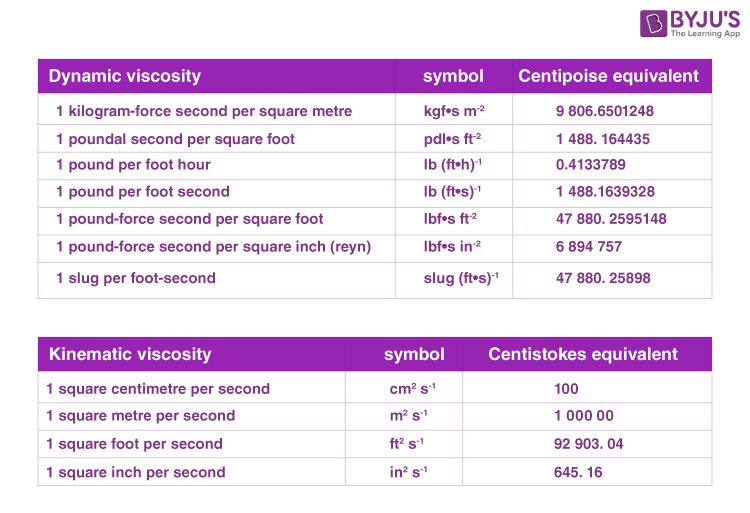# Unit of Viscosity

## What is Viscosity?

Viscosity is defined as the quantity that represents a fluid’s resistance to flow. To put it in simpler terms, viscosity is the friction between the fluid molecules. We usually relate to the viscosity of a liquid by measuring the “thickness”. For example, we can state that honey is “thicker” (meaning a higher viscosity) than normal water.

Viscosity is represented by the symbol η “eta”, and the use of the Greek letter mu (μ) is also common. Furthermore, viscosity is also known as dynamic viscosity or absolute viscosity. Apart from this, we also deal with terms like kinematic viscosity or momentum diffusivity.  The main difference between kinematic and dynamic viscosity is that dynamic viscosity is the measurement of a fluid’s internal resistance to the flow. In contrast, kinematic viscosity is the ratio of dynamic viscosity to density. Now that we have learned some viscosity information let’s study the unit of viscosity below.

 Unit of Viscosity SI Unit Pascal seconds (Pa·s) or kg·m−1·s−1 CGS Unit Poise (P) Unit of Kinematic Viscosity Si Unit square metre per second or m2/s CGS Unit Stokes (St)

### SI Unit of Viscosity

• The SI unit of viscosity is the pascal second (Pa·s) or kg·m−1·s−1.
• The SI unit of kinematic viscosity is square metre per second or m2/s.

### CGS Unit of Viscosity

• The cgs unit of viscosity is poise (P), which has been used in honour of French physiologist Jean Léonard Marie Poiseuille. It is also commonly used as centipoise (cP), particularly in ASTM standards.
• The cgs unit for kinematic viscosity is stoke (St) and has been named after Irish mathematician and physicist Sir George Gabriel Stokes. Sometimes the unit centistokes (cSt) is also used.

#### Other Common Viscosity Units

Some non-standard units include the Reyn, which is a British unit of dynamic viscosity.

Earlier, kinematic viscosity was measured using the Saybolt viscometer, expressing the units in Saybolt universal seconds (SUS). Other abbreviations such as SUV (Saybolt universal viscosity) or SSU (Saybolt seconds universal) are sometimes used.Related Articles:

Q1

### State Newton’s law of viscosity.

Newton’s law of viscosity states that shear stress between the adjacent layers of the fluid is proportional to the velocity gradients between the two layers.

Q2

### What will be the viscosity of fluid when the fluid is at rest?

The viscosity of fluid when it is at rest will be 1 Poise. Viscosity is not dependent on the state of the fluid. Therefore, if the fluid is at rest or in motion, 1 Poise will remain constant.

Q3

### Mention the factors on which viscosity depends.

Following are the factors on which viscosity depends:

• Area of contact between the two adjacent layers
• The velocity difference between the two adjacent layers
• Distance between the two adjacent layers
Q4

### Convert 1 Poise into N.s.m-2.

$$\begin{array}{l}1\;Poise= 1\;dyne\;s\;cm^{-2}=\frac{1}{10}N\;s\;m^{-2}\end{array}$$
Q5

### Give examples of Newtonian fluids.

Water, honey, and organic solvents are examples of Newtonian fluids.

Stay tuned with BYJU’S to learn more interesting Physics topics with the help of engaging video lessons.

Test your knowledge on Unit Of Viscosity